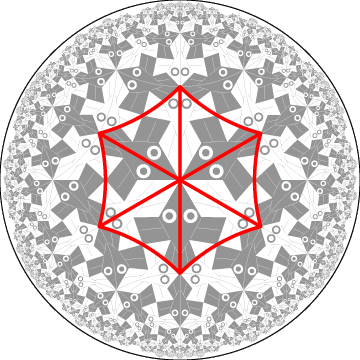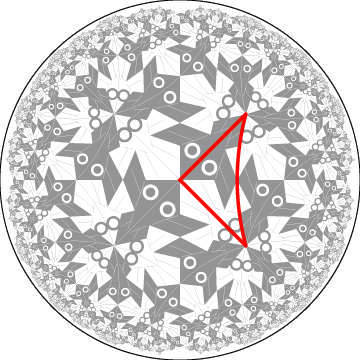## 4. Transformation of a Pattern

The basic version of the computer program that performs the transformation requires that the motif be contained in one of the p isosceles triangles formed by the radii of a p-gon. Figure 3 below shows the isosceles triangles within a 6-gon of {6,4} that is the basis of Escher's Circle Limit I (shown in gray). A natural motif in Circle Limit I is composed of a black half-fish and an adjoining white half-fish, however such a motif has part of a white fish fin protruding outside its isosceles triangle. This motif can be modified to the required form by clipping off the protruding part and "gluing" it back between the tail and the back edge of the fin of the black fish.Figure 3: Six isosceles triangles superimposed on Escher's Circle Limit I pattern.

The basic transformation process makes use of the Klein model of hyperbolic geometry. As with the Poincaré model, the points are interior points of a bounding circle, but the hyperbolic lines are represented by chords. We let I denote the isomorphism that maps the Poincaré model to the Klein model. Then I maps a centered p-gon with its isosceles triangles to a regular p-sided polygon which also contains corresponding isosceles triangles. Different tessellations {p,q} produce different isosceles triangles in the Klein model, but an isosceles triangle from {p,q} can be mapped onto an isosceles triangle from {p',q'} by a simple (Euclidean) differential scaling, since those isosceles "Klein" triangles are represented by isosceles Euclidean triangles. Thus the transformation from a {p,q} pattern to a {p',q'} pattern can be accomplished by
(1) applying I to a motif in an isosceles triangle of {p,q},
(2) applying the differential scaling to that transformed triangle, and finally
(3) applying the inverse of  I to the re-scaled triangle containing the motif.
The entire pattern can then be formed by replicating the transformed motif. Replication algorithms are discussed in Dunham (1986a) and Dunham (1986b). Figure 4 shows the result of transforming the Circle Limit I pattern to a {4,6} pattern - with a transformed isosceles triangle superimposed.Figure 4: A transformed Circle Limit I based on {4,6} showing one isosceles triangle.

Using similar techniques, another program has been written to transform isosceles Euclidean triangles to isosceles hyperbolic triangles, and thus Euclidean Escher patterns (of which there are many) can be transformed to hyperbolic patterns.

 INDEX NEXT